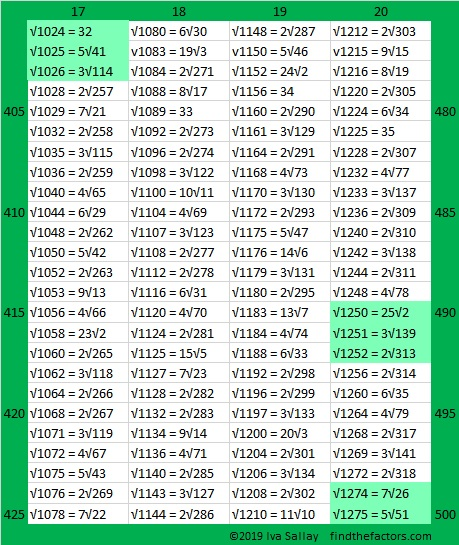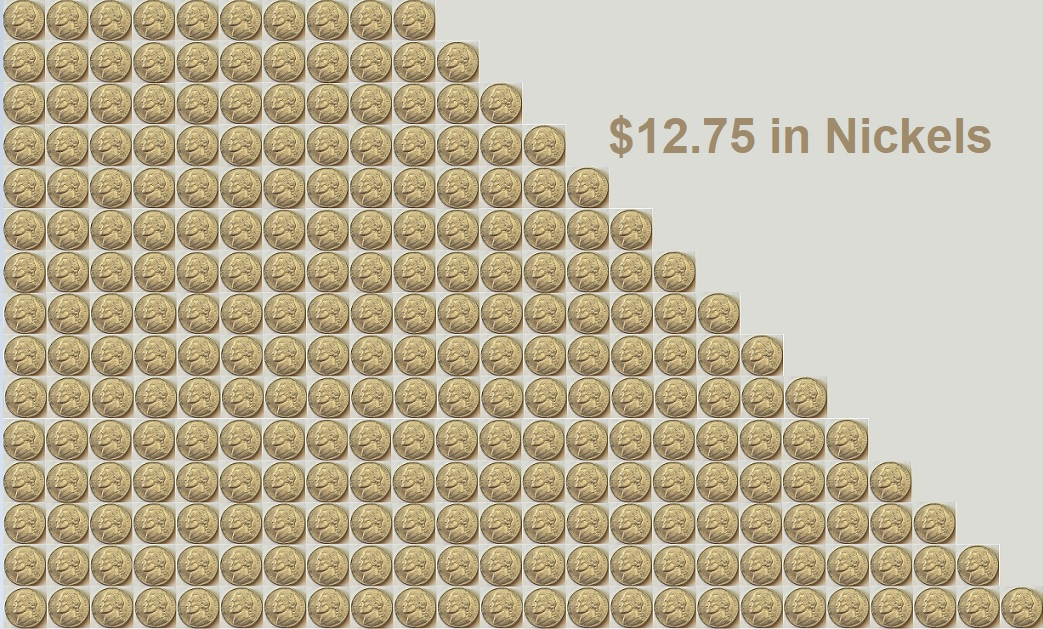# 1275 is the 50th Triangular Number AND the 500th Number Whose Square Root Can Be Simplified

I found two reasons to celebrate the number 1275: It is the 50th Triangular number, and it is the 500th number whose square root can be simplified.

First I’ll celebrate its square root by listing the 401st to the 500th numbers with simplifiable square roots. Having three or more simplifiable square roots in a row doesn’t happen that often, so I like to highlight them when it happens. 1274 and 1275 are highlighted because 1276 also has a square root that can be simplified:If you’re wondering what are the first 400 numbers with simplifiable square roots, you can click on the graphics below that will give you 100 at a time:

Now to celebrate that 1275 is the 50th triangular number, I’ve arranged \$12.75 in a triangle:1275 can also be evenly divided by 5, and 25, in other words, by nickels and quarters!

Nickels won’t make a triangle but they can form a trapezoid. Here’s how I made this one: 1275 ÷ 5 = 255 which is 300 (the 24th triangular number) minus 45 (the 9th triangular number). Thus we can make \$12.75 by arranging 255 nickels in a trapezoid with a top base of 10, a bottom base of 24, and a height of 15.We can also use quarters to make a trapezoid. Here’s what I did: 1275 ÷ 25 = 51 which is 66 (the 11th triangular number) minus 15 (the 5th triangular number). Thus, \$12.75 can be made by arranging 51 quarters in a trapezoid with a top base of 6, a bottom base of 11, and a height of 6.Can you find any rectangular ways to arrange the coins to total \$12.75?

Here’s a little more about the number 1275:

• 1275 is a composite number.
• Prime factorization: 1275 = 3 × 5 × 5 × 17, which can be written 1275 = 3 × 5² × 17
• The exponents in the prime factorization are 1, 2, and 1. Adding one to each and multiplying we get (1 + 1)(2 + 1)(1 + 1) = 2 × 3 × 2 = 12. Therefore 1275 has exactly 12 factors.
• Factors of 1275: 1, 3, 5, 15, 17, 25, 51, 75, 85, 255, 425, 1275
• Factor pairs: 1275 = 1 × 1275, 3 × 425, 5 × 255, 15 × 85, 17 × 75, or 25 × 51,
• Taking the factor pair with the largest square number factor, we get √1275 = (√25)(√51) = 5√51 ≈ 35.707141275 is the hypotenuse of SEVEN Pythagorean triples:
195-1260-1275 which is 15 times (13-84-85)
261-1248-1275 which is 3 times (87-416-425)
357-1224-1275 which is (7-24-25) times 51
540-1155-1275 which is 15 times (36-77-85)
600-1125-1275 which is (8-15-17) times 75
765-1020-1275 which is (3-4-5) times 255
891-912-1275 which is 3 times (297-304-425)

This site uses Akismet to reduce spam. Learn how your comment data is processed.